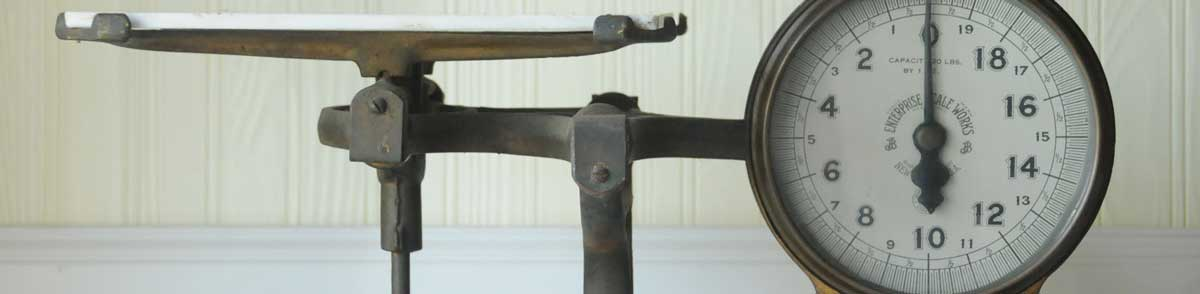# Kilograms to Calories Conversion

Enter the weight in kilograms below to get the value converted to calories.

Results in Calories:1 kg = 7,716.179176 kcal

## How to Convert Kilograms to CaloriesTo convert a kilogram measurement to a calorie measurement, multiply the weight by the conversion ratio.

Since one kilogram of body fat is equal to 7,716.179176 calories, you can use this simple formula to convert:

calories = kilograms × 7,716.179176

The weight in calories is equal to the kilograms multiplied by 7,716.179176.

For example, here's how to convert 5 kilograms to calories using the formula above.
5 kg = (5 × 7,716.179176) = 38,580.895882 kcal

### How Many Calories are in a Kilogram of Body Fat?

There are 7,716.179176 calories in a kilogram of body fat, which is why we use this value in the formula above.

1 kg = 7,716.179176 kcal

Kilograms are a unit of weight, and calories are a unit of energy. Keep reading to learn more about each unit of measure.

## Kilograms

The definition of the kilogram changed in 2019. One kilogram was previously equal to the mass of the platinum-iridium bar, known as the International Prototype of the Kilogram, which was stored in Sèvres, France.

The 2019 SI brochure now defines the kilogram using the Planck constant, and it is defined using the meter and second. It is roughly equal to the mass of 1,000 cubic centimeters of water.

The kilogram, or kilogramme, is the SI base unit for weight and is also a multiple of the gram. In the metric system, "kilo" is the prefix for 103. Kilograms can be abbreviated as kg; for example, 1 kilogram can be written as 1 kg.

## Calories

Converting calories is actually a conversion of energy burned by the body performing work rather than actual weight. When measuring the energy content in foods, the large calorie, or kilocalorie, is used, but it's often still just called a "calorie."

One calorie (large calorie) is equal to the heat needed to raise the temperature of one kilogram of water by 1 degree Celsius. You'll need to burn 3,500 calories more than you eat to lose one pound of weight.

Calories can be abbreviated as kcal; for example, 1 calorie can be written as 1 kcal.

## Kilogram to Calorie Conversion Table

Kilogram measurements converted to calories
Kilograms Calories
0.001 kg 7.7162 kcal
0.002 kg 15.43 kcal
0.003 kg 23.15 kcal
0.004 kg 30.86 kcal
0.005 kg 38.58 kcal
0.006 kg 46.3 kcal
0.007 kg 54.01 kcal
0.008 kg 61.73 kcal
0.009 kg 69.45 kcal
0.01 kg 77.16 kcal
0.02 kg 154.32 kcal
0.03 kg 231.49 kcal
0.04 kg 308.65 kcal
0.05 kg 385.81 kcal
0.06 kg 462.97 kcal
0.07 kg 540.13 kcal
0.08 kg 617.29 kcal
0.09 kg 694.46 kcal
0.1 kg 771.62 kcal
0.2 kg 1,543 kcal
0.3 kg 2,315 kcal
0.4 kg 3,086 kcal
0.5 kg 3,858 kcal
0.6 kg 4,630 kcal
0.7 kg 5,401 kcal
0.8 kg 6,173 kcal
0.9 kg 6,945 kcal
1 kg 7,716 kcal

## References

1. International Bureau of Weights and Measures, The International System of Units, 9th Edition, 2019, https://www.bipm.org/documents/20126/41483022/SI-Brochure-9-EN.pdf
2. Brazier, Yvette, How many calories do you need?, Medical News Today, https://www.medicalnewstoday.com/articles/263028
3. U.S. Department of Agriculture, I want to lose a pound of weight. How many calories do I need to burn?, https://www.nal.usda.gov/fnic/i-want-lose-pound-weight-how-many-calories-do-i-need-burn